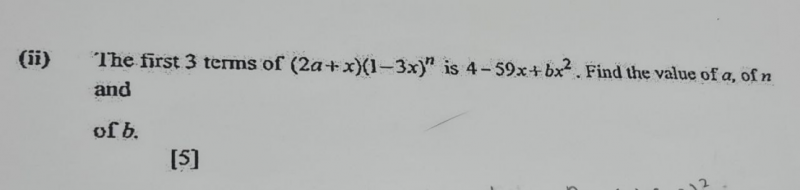# QuestionHi, can anyone help with this binomial question please? Thank you

(2a+x)(1-3x)n                                                                                   =  4 -59x + bx2 + …..

Using the Binomial expansion of:

(1+x)n =  1 + nx + n!/[(n-2)! 2! ] x2 + ………    replacing x with (-3x)

(2a+x) { 1 + n(-3x) + n!/[(n-2)! 2! ] (-3x) + ……..}  = 4 -59x + bx2 + …..

2a(6an)x  +  n!/[(n-2)! 2! ](2a)(9x2) + (1) x – (3n) x2 + …….    459x + bx + …….

2a = 4 => a = 2

Comparing coefficient of x and substituting a =2,

6an – 1 = 59  => 12n = 60   => n = 5

Comparing coefficient of x2 and substituting n = 5, a =2,

b = (5!/2!3!) (18×2) – 15 = 360 – 15 = 345

Therefore, a = 2, n = 5 and b = 345